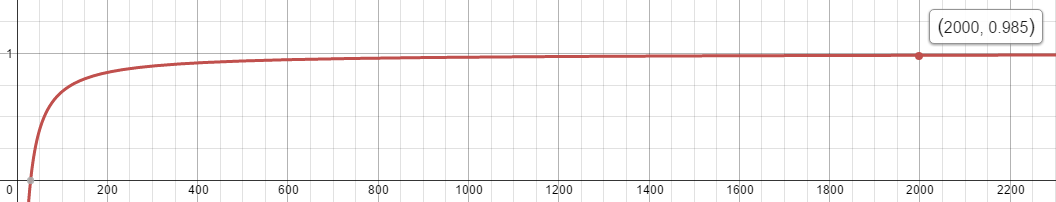# Expressing relationship between two-numbers as a percentage based on their quotient

### Or, numbers are fun and here is a way to wow someone

#### March 18, 2016

I love finding relationships in number-sets. A favorite memory from high school deals with a game we would play in Algebra class. The teacher would give us a set of numbers and one target number. The goal was to use the set of numbers and arithmetic operations to get to the target. Here is an example:

Set: $${1, 2, 4, 6}$$

Target: $$49$$

A couple solutions: $$[1 + (2 * 4) * 6], [1 + 2 * (4 * 6)]$$

You can find problems like this in a handy desk calendar (non-affiliate link).

Fast forward several years and I started thinking about age as a percentage between a parent and child. It seemed interesting that a child is half the age of their parent when the child is the same age as the parent when the child is born. At first, I thought this was a fluke of the number-world, but gave it more thought, given both child and parent age at the same numerical-rate. I was interested in finding a general-purpose equation–one that could be applied at any age and came up with: $$a = (b + a)c$$, where $$a$$ is the child's age, $$b$$ is the age of the parent when the child is born, and $$c$$ is the relationship between both as a percentage. Let us go through an example:

My Dad is thirty years old when I am born. When I am ten, what percentage of my Dad's age am I?

Solution:

• $$10 = (30 + 10)c$$

• $$10 = 40c$$

• Divide both sides by 40

• $$c = 10 / 40$$

• $$c = 0.25$$ or 25%

What I really want to do, though, is find age based on percentage. Now, the question becomes what is my age when I am twenty-five percent of my Dad's age? Taking the original equation, we can work to get $$a$$ on one-side:

• $$a = (b + a)c$$

• Distribute the right-side

• $$a = bc + ac$$

• Subtract $$ac$$ from both-sides

• $$a - ac = bc$$

• Factor $$a$$ from the left-side

• $$a(1 - c) = bc$$

• Divide both sides by $$(1 - c)$$

• $$a = (bc) / (1 - c)$$

And plugging our numbers into the equation:

• $$a = (30 * 0.25 ) / (1 - 0.25)$$

• $$a = 7.5 / 0.75$$

• $$a = 10$$

But, we can do even better–we can also answer the question what is the age of my Dad when I am twenty-five percent of his age? Again, looking at the original equation, we can create a new variable $$d$$ and put it in place of $$(b + a)$$:

• $$a = dc$$

• Divide both sides by $$c$$

• $$d = a / c$$

We already solved for $$a$$, so we can put it into the equation:

• $$d = ((cb) / (1 - c))/c$$

• Let us convert the outer division problem into multiplication for better readability

• $$d = ((cb) / (1 - c))(1 / c)$$

• Multiply both numerators and denominators

• $$d = (cb) / (c - c^2)$$

• Factor $$c$$ from the denominator

• $$d = (cb) / (c(1 - c))$$

• We can now remove $$c$$ from both numerator and denominator

• $$d = b / (1 - c)$$

And plugging our numbers into this equation:

• $$d = 30 / (1 - 0.25)$$

• $$d = 30 / 0.75$$

• $$d = 40$$

Of course, these equations are not limited to comparing age. They can be applied to any two-numbers. The key is to represent $$b$$ relative to zero. So, if $$a$$ is not zero, then $$b$$ becomes $$b-a$$. Keep in mind, however, $$c$$ cannot be one-hundred percent. This leads to division by zero. Lastly, for some extra visual flaire, we can graph the results using $$x = 30 / (1 - y)$$. Keeping with our theme, this plots my Dad's age on the x-axis with my percentage (in decimal-form) of his age on the y-axis. Notice the asymptotic-nature of the line:Graphs plotted using https://www.desmos.com/calculator.